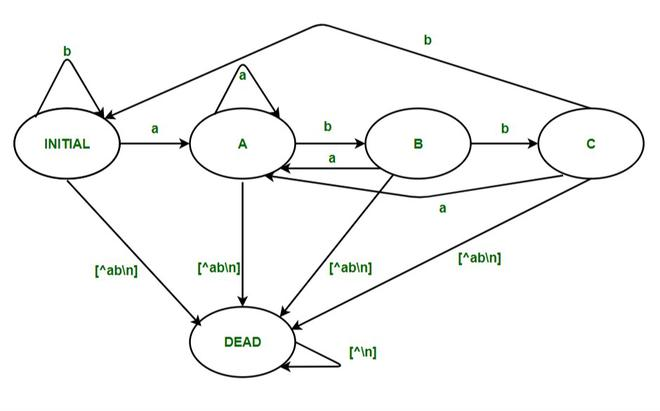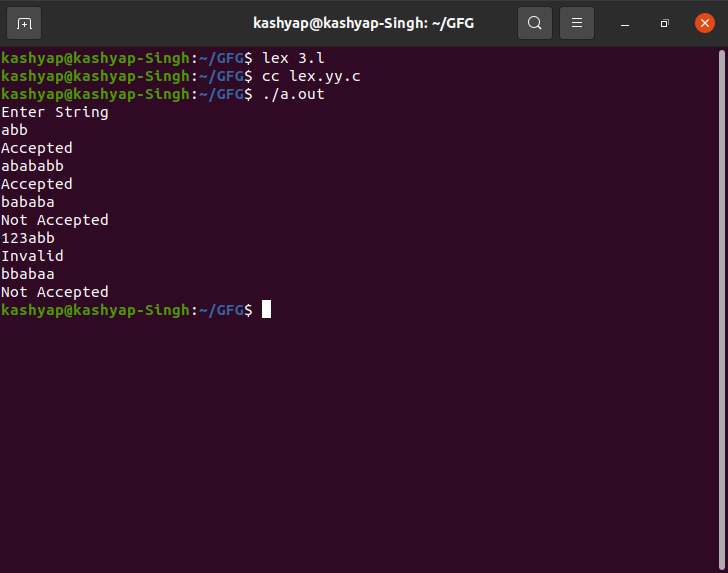Related Articles

# LEX Code that accepts the string ending with ‘abb’ over input alphabet {a,b}

• Difficulty Level : Hard
• Last Updated : 23 Jul, 2021

In this article, we will discuss the LEX Code that accepts the string ending with ‘abb’ over input alphabet  {a,b} and will see the implementation using LEX code and will understand the approach. Let’s discuss it one by one.

Problem Overview :
LEX Code that accepts the string ending with ‘abb’ over input alphabet  {a,b}.

Example –

```Input  : abb
Output : Accepted

Input  : abababb
Output : Accepted

Input  : 23ab
Output : Invalid

Input  : ab345
Output : Invalid

Input  : bbabaa
Output : Not Accepted```

Approach :
LEX provides us with an INITIAL state by default. So to make a DFA, use this as the initial state of the DFA. We define four more states: A, B, C, and DEAD, where the DEAD state would be used if encountering a wrong or invalid input. When the user inputs an invalid character, move to DEAD state, and then print “Invalid”. If the input string ends at C then display the message “Accepted”. Else if the input string ends at state INITIAL, A, or B then displays the message “Not Accepted”.Note –
To compile the lex program we need to have a Unix system that has flex installed into it. Then we need to save the file with the .l extension.

Example –

`filename.l`

Then after saving the program closes the lex file and then open the terminal and write the following commands as follows.

```lex filename.l
cc lex.yy.c
./a.out```

Implementation- LEX Code :

```%{
%}

// not accepted state after visiting A
%%
<INITIAL>a BEGIN A;
<INITIAL>b BEGIN INITIAL;
<INITIAL>\n BEGIN INITIAL; {printf("Not Accepted\n");}

// not accepted state after visiting A and B state
<A>a BEGIN A;
<A>b BEGIN B;
<A>\n BEGIN INITIAL; {printf("Not Accepted\n");}

// // not accepted state after visiting A and C state
<B>a BEGIN A;
<B>b BEGIN C;
<B>\n BEGIN INITIAL; {printf("Not Accepted\n");}

// Accepted case
<C>a BEGIN A;
<C>b BEGIN INITIAL;
<C>\n BEGIN INITIAL; {printf("Accepted\n");}

// Invalid Case

%%
// yywrap method
int yywrap()
{
return 1;
}

// main method
int main()
{
printf("Enter String\n");
// called yylex
yylex();
return 0;
}```

Output :Attention reader! Don’t stop learning now. Get hold of all the important CS Theory concepts for SDE interviews with the CS Theory Course at a student-friendly price and become industry ready.

My Personal Notes arrow_drop_up